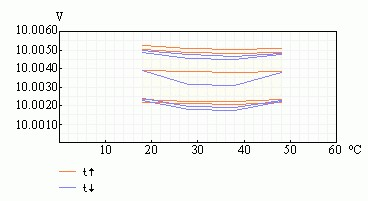# 10V High-Stability Voltage Source ModelFig .1 Highly stable source of voltage schematic

Experimental studies of exemplary scheme of a highly stable source of voltage (Fig. 1) in the temperature range from -18 ° C to 50 ° C show that the temperature error of less than ± 0.001% / ° C, temporal drift no more than ± 0.005% per day. Source has a small thermal hysteresis (Fig. 2).Fig. 2 temperature characteristic of source

Parts list :

• R1, R3 =1K
• R2 = 15K
• R4 = 6K8
• R5 = 2K2
• R6,R10 = 110 ohm
• R7 = 11 ohm
• R8 = 820 ohm
• R9 = 3K3
• C1,C2 = 10nF
• D1 = D818E

To ensure high stability in the scheme used the method of heating the crystal heating and temperature monitoring. To study the selected type of transistor assembly 1NT251 with Formula. Transistor Q2 is used to heat the crystal, and Q4 – a temperature sensor. Zener diode D1 – D818E. The operating mode is set for not more than 1 minute.

Power supply is an electronic device that supplies electric energy to an electrical load. The primary function of a power supply is to convert one form of electrical energy to another and, as a result, power supplies are sometimes referred to as electric power converters. Some power supplies are discrete, stand-alone devices, whereas others are built into larger devices along with their loads. Examples of the latter include power supplies found in desktop computers and consumer electronics devices.

## Related Post "10V High-Stability Voltage Source Model"

Comments are closed.